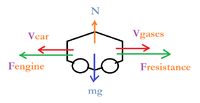# Motion in a Straight Road

#### nasi112

##### Full Member
A car of mass $$\displaystyle 600 \ kg$$ is driven along a straight road. The resistance to motion of the car is $$\displaystyle kv^2$$ Newtons, where $$\displaystyle v \ m/s$$ is the velocity of the car at time $$\displaystyle t$$ seconds and $$\displaystyle k$$ is a constant. The engine of the car is producing $$\displaystyle 8 \ kW$$ of output power.

A.
i. Draw a free body diagram of the system.
ii. Derive the differential equation of motion of the vehicle, and describe it in the form:
$$\displaystyle \frac{dv}{dt} + Av^2 - \frac{B}{v} = 0$$
Where $$\displaystyle A$$ and $$\displaystyle B$$ are constants to be found.

B. When the velocity of the car is $$\displaystyle 20 \ m/s$$, the engine is turned off.

i. Draw a free body diagram of the system.
ii. Derive the differential equation of motion of the car, and describe it in the form:
$$\displaystyle \frac{dv}{dt} + Av^2 = 0$$
Where $$\displaystyle A$$ is a constant to be found.
iii. Find, in term of $$\displaystyle k$$, an expression for the velocity of the car. Make sure your expression is in the form $$\displaystyle v = f(t)$$

This is what I did for $$\displaystyle A$$I am not sure if this is the correct free body diagram. Can anyone help me, please?

Now, I will try to derive the differential equation.

$$\displaystyle F_e - F_R = ma = m\frac{dv}{dt}$$

$$\displaystyle m\frac{dv}{dt} + kv^2 - F_e = 0$$

Now I will use the output power

$$\displaystyle F_e = \frac{P}{v}$$

$$\displaystyle m\frac{dv}{dt} + kv^2 - \frac{P}{v} = 0$$

$$\displaystyle \frac{dv}{dt} + \frac{kv^2}{m} - \frac{P}{mv} = 0$$

Is this a correct derivation? Please, let me know if I did something wrong in the derivation or in the free body diagram.

I also noticed that if I want to solve this differential equation with separable method, it gives a very complicated solution while wolfram can solve it easily $$\displaystyle v(t) = -Atv^2 + \frac{Bt}{y} + c_1$$, if I let $$\displaystyle A = \frac{k}{m}$$ and $$\displaystyle B = \frac{P}{m}$$

Why is that?

#### Attachments

• 9.6 KB Views: 0